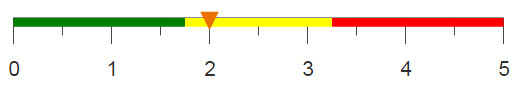# Performing a time comparison analysis

Comparing aggregated data for two different time periods can help to identify patterns and changes in data.

## Difficulty## Objective

You want to perform an analysis that compares the data from one time period to a previous time period. For instance, retailers often want to compare this week’s sales to last week’s sales. Another example is comparing the average temperature of July this year to the average temperature of July last year. In order to do this, the values for each time period must be in the same row but in different columns. Often, especially in time-ordered data sets, the values you want to compare are located in the same column but not in the same row. One solution is to use the 1010data function g_rshift(G;S;O;X;N) to create a new column that contains the values shifted by the time period required by the analysis. Alternatively, you can compute a weighted sum in a tabulation using reference columns, one for each time period, to place the desired values in new columns in the same row.

## Solution

```<base table="pub.doc.retail.altseg.sales_detail_transid"/>
<willbe name="month" label="Month" value="month(trans_date)"/>
<willbe name="year" label="Year" value="year(trans_date)"
format="type:nocommas"/>
<tabu label="Tabulation" breaks="year,month">
<break col="year" sort="up"/>
<break col="month" sort="up"/>
<tcol source="xsales" fun="sum" name="sum" label="Sum of Sales"/>
</tabu>
<willbe name="last_year" label="Last Years Sum"
value="g_rshift(month;;;sum;-1)" format="dec:2"/>
<willbe name="yoy" label="YOY" value="sum-last_year" format="dec:2"/>
<willbe name="yoy_perc" label="YOY Percentage" value="yoy/last_year"
format="type:pct;dec:2"/>```

## Alternate solution

```<base table="pub.demo.weather.wunderground.observed_daily"/>
<sel value="meantempi<150"/>
<sel value="meantempi>-40"/>
<colord cols="zipcode,date,meantempm,meantempi"/>
<sel value="year(date)=2014 2015"/>
<willbe name="month" label="Month" value="month(date)"/>
<willbe name="year" label="Year" value="year(date)" format="type:nocommas"/>
<willbe name="mean" value="meantempi" format="type:num"/>
<tabu label="Tabulation on Observed Daily" breaks="month,year">
<tcol source="mean" fun="avg" name="mean_m"
label="Mean Monthly Temperature (F)"/>
</tabu>
<willbe name="fy2014" label="FY2014" value="year=2014"/>
<willbe name="fy2015" label="FY2015" value="year=2015"/>
<tabu label="YOY Mean Monthly Temperature" breaks="month">
<tcol source="mean_m" fun="sumwd" name="mean2014" weight="fy2014"
label="2014 Mean Monthly`Temperature"/>
<tcol source="mean_m" fun="sumwd" name="mean2015" weight="fy2015"
label="2015 Mean Monthly`Temperature"/>
</tabu>
<willbe name="yoy" label="YOY" value="mean2015-mean2014" format="dec:2"/>
<willbe name="yoy_perc" label="YOY Percentage" value="yoy/mean2014"
format="type:pct;dec:2"/>
<sort col="month"/>```

## Discussion

Performing a time comparison analysis allows you to examine how your data changes from one time period to another. Additionally, the summarized values for each time period should be displayed in adjacent columns to allow for easy comparison between the two.

This recipe presents two methods. The most efficient way is to use the 1010data function g_rshift(G;S;O;X;N) to shift rows according to time period. In the primary solution, this function is used to create a new column that, along with additional columns, can then be used to determine the percent loss or gain from one year to the next. The second solution utilizes the dot-product (or weighted sum) method to calculate the year-over-year change for each month in the data set.

A key component in either method is having a date column with dates stored in the standard integer format of YYYYMMDD that 1010data uses. If the dates are not stored in this format or if they are stored as text values, it is essential to transform them into the correct format. For assistance in performing this transformation, see the recipe titled Transforming dates from text to integers.

In the first solution, two new columns representing the month and year are used in a tabulation over each month/year combination. (Note that the sample data set, pub.doc.retail.salesdetail, only contains information for January of 2014 and 2015.) After the tabulation, the g_rshift(G;S;O;X;N) function is used to shift the rows in the worksheet so that the same month from both years in the analysis are in adjacent rows. Once the aggregations are in the same row, calculating the difference between them, and ultimately the percentage change, is simple.

The second solution, which uses the weighted sum approach is similar, but instead of shifting rows, a column containing a boolean flag is created for each year in the analysis. After the monthly values are calculated, this flag column is then used as the reference column, which acts as a weight for the dot product in the final tabulation. Again, with the values now in adjacent rows, computing the percent loss or gain from one year to the next is straightforward.

## Further reading

If you would like to learn more about the functions and operations discussed in this recipe, click on the links below:

g_rshift(G;S;O;X;N)

<tabu>